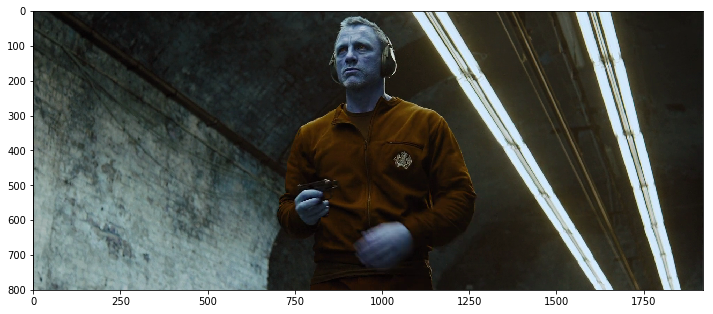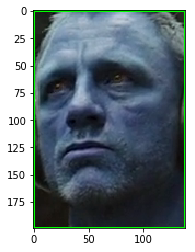# Generate face Embeddings to tag local movies, videos, photos-collection

## Overview:

FaceEmbeddings module is trained to generate Embeddings(a 512-d float32 vector), such that embeddings for the same face lies closer in the 512 dimensional space .

This module implements a siamese network which is trained with pairs of `positive-positive` and `positive-negative` cropped faces. Selection of such pairs directly influences the quality of embeddings generated by model. While `positive-negative` pair is supposed to contain faces of similar but, NOT SAME persons and hence forces the model to learn higher semantic features necessary to distinguish two different faces. `positive-positive` pairs on the other hand, are supposed to contain face of same person in diverse set of conditions including lighting, angle etc. Both pairs force the network to learn actual semantic features which would not have been possible with a single type of pair, such models works really well for real-life scenarios.

But such models are generally sensitive to alignment of `cropped-face` being passed to generate final embedding, and are generally coupled with a `face-alignment` procedure. It involves linearly transforming the cropped face based on the detected `facial-landmarks` which is then passed to the network to generate final embeddings.

In this tutorial, we would be using `faceEmbeddings` module to generate `embeddings` for a desired face( template embedding) and would then use this `template-embedding` to compare generated embeddings (`target-embeddings`) in the following frames/images. Comparison step basically measures the direct-distance( `Eucledian-norm` ) b/w any two such embeddings( vectors ). This Module just expects `BGR` raw-data as input, it takes care of `face-detection` and `face-alignment` steps before generating final `embeddings`.

This can be used easily to tag your own `photo-collection` locally and make it searchable.

``````import numpy as np
import matplotlib.pyplot as plt
``````
``````import faceEmbeddingsPipeline_python_module as pipeline
``````
``````#initialize our module.
``````

## Encode a template:

We would be encoding a face for the person we want to detect in all the following frames. Choose any image of any resolution with a single face in the frame. We can even choose an image with more than one face in the image, we just would have to select the corresponding Embeddings.

Let us try to encode `Daniel Craig` from the `Skyfall` movie.

``````template = cv2.imread("../../data/skyfall_3.png")  # [BGR uint8 ] BGR format is preferred over RGB data.
print("Resolution: {}".format(template.shape))

#display the image
plt.figure(figsize=(12,12))
plt.imshow(template)
``````
``````Resolution: (800, 1920, 3)

<matplotlib.image.AxesImage at 0x1790942ba90>
``````## Detect the face and generate Embedding:

We would now detect desired facial bounding-box and corresponding Embeddings on given template image. Generated embeddings would then be treated as `template Embedding` and would be compared with embeddings generated from target frames/images.

Module `detect_embedding` function returns the generated `embeddings` and corresponding `facial bounding boxes`.

``````bounding_boxes,embeddings = pipeline.detect_embedding(template,conf_threshold=0.85)
``````
``````print(bounding_boxes.shape)
print(embeddings.shape)
print(bounding_boxes)  #x1,y1,x2,y2 format (left,top) (right,bottom)
``````
``````(1, 4)
(1, 512)
[ 864.   45. 1003.  244.]
``````
``````for i in range(bounding_boxes.shape):
x1,y1,x2,y2 = bounding_boxes[i]
cv2.rectangle(template,(int(x1),int(y1)),(int(x2),int(y2)),(0,255,0),2)

plt.figure(figsize=(12,12))
plt.imshow(template)
``````
``````<matplotlib.image.AxesImage at 0x179094a1358>
````````````plt.imshow(template[int(y1):int(y2),int(x1):int(x2),:])
``````
``````<matplotlib.image.AxesImage at 0x1790950b160>
``````If there are more than one face detected user can choose to select a single face by displaying detected facial bounding boxes and choosing one. Since in our case only a single face is present, we would select the corresponding embedding as shown below.

``````idx = 0  # point it to desired one, in case there are more than one faces.
template_embedding = embeddings[idx:idx+1,:]
``````

### Writing a simple function to encode a face given an image raw-data.

``````#writing a simple routine to return template_embedding given an image with a single face.
def encode_template(template_path:str,conf_threshold:float=0.85):
bboxes,embeddings = pipeline.detect_embedding(frame,conf_threshold=conf_threshold)
assert bboxes.shape == 1, "expected a single face in given frame but got {}".format(bboxes.shape)
return embeddings[0:1,:]
``````

## How to Compare Embeddings:

We would be calculating square of direct distance if embeddings were to be lying in a `512D` space. i.e square of `eucledian distance/Norm`.

Empirical threshold can be taken as `1.32`.

``````def compare_embeddings(template_embedding,target_embeddings):
assert template_embedding.shape == (1,512)
distance = np.sum(np.square(target_embeddings-template_embedding),axis=1)
return distance
``````
``````compare_embeddings(template_embedding,template_embedding)
``````
``````array([0.], dtype=float32)
``````

## Now start generating Embeddings on the images/videos of your choice.

``````EMPIRICAL_THRESHOLD = 1.32 # set it to lower, if accurate facial-recognition is a priority.

bounding_boxes,embeddings = pipeline.detect_embedding(frame,conf_threshold=0.75)
distance_array = compare_embeddings(template_embedding,embeddings)
print(distance_array)
indices = (distance_array < EMPIRICAL_THRESHOLD).astype("uint8")

for count in range(bounding_boxes.shape):
x1,y1,x2,y2 = bounding_boxes[count]
color = (0,0,255)
if indices[count] == 1:
color = (0,255,0)
cv2.rectangle(frame,(int(x1),int(y1)),(int(x2),int(y2)),color,2)

plt.figure(figsize=(12,12))
plt.imshow(frame[:,:,::-1])
``````
``````[0.8482303 1.7437494 2.0798588 2.0018718]

<matplotlib.image.AxesImage at 0x179095d3e10>
``````## API used:

Face Embedding SDK

``````
``````